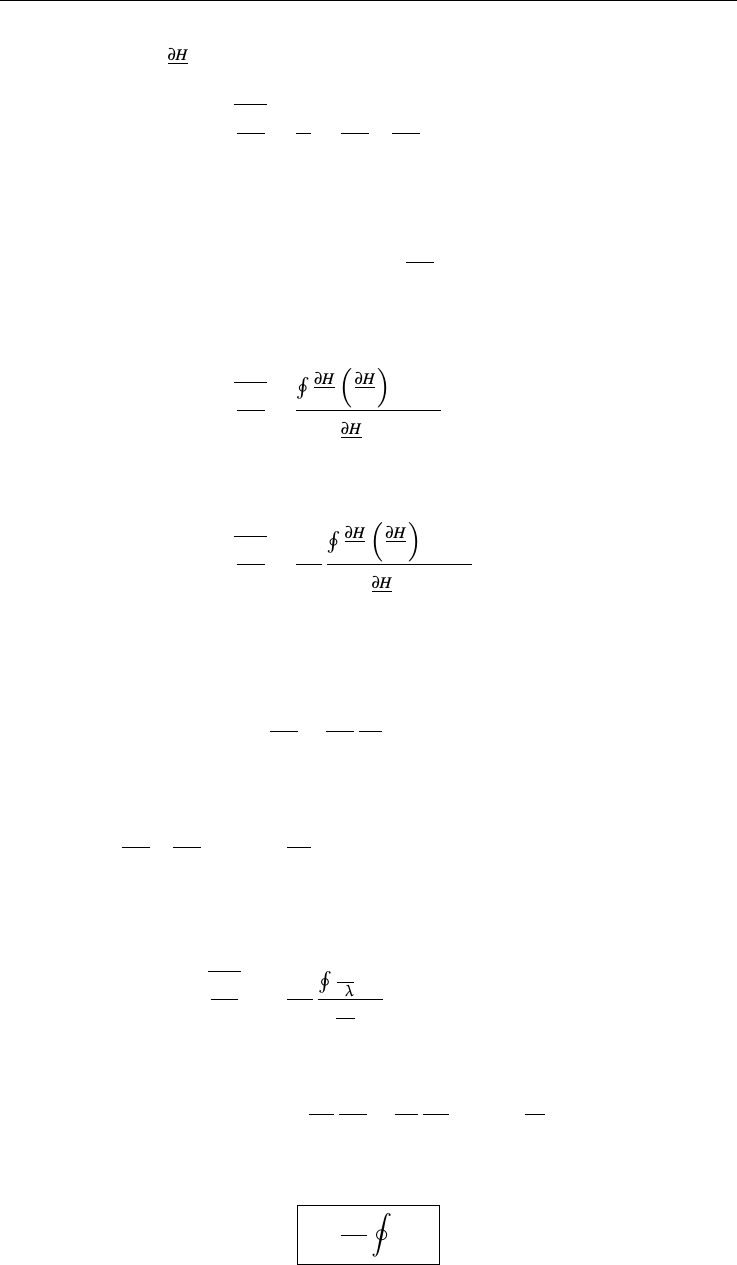Study Guides (400,000)
US (230,000)
CU-Boulder (1,000)
PHYS (60)
Final

# PHYS 4150 Lecture 9: plasma_9Exam

Department
Physics
Course Code
PHYS 4150
Professor
Sascha Kempf
Study Guide
Final

This preview shows pages 1-2. to view the full 6 pages of the document.PHYS4150 — P L A S M A P H Y S I C S
l e c t u r e 9 - m a g n e t i c m i r r o r m a c h i n e s ,a d i a b a t i c
m o m e n t s ,t r a p p i n g
Sascha Kempf
Fall 2019
1a d i a b at i c i n va r i a n t s
The presence of adiabatic invariants is actually a common phenomenon, which has
been studied extensively in classical mechanics. Here we follow Landau & Lifschitz
and consider a one-dimensional ﬁnite motion, where
λ
is a parameter describing a
very slow change of the system. Here, slow means slow compared to the period
T
of
the cyclic motion, i.e.
T˙
λλ
. Now, because
λ
is slowly changing, so is the energy
E
of the system, where
˙
E˙
λ
. This implies that the change of energy is a function
of λ, from what follows that there is a combination of Eand λ, a so-called adiabatic
invariant, which remains constant.
Now let
H(p,q;λ)
be the Hamiltonian of such a system, where again
λ
is the
parameter characterizing the slow change. Then,
dE
dt=H
t=H
λ
dλ
dt.
Now we average over one cycle
T
and assume that
˙
λ
does not change on this time
scale
dE
dt=dλ
dt
H
λ.
Now,
H
λ=1
T
T
Z
0
H
λdt,
1

Only pages 1-2 are available for preview. Some parts have been intentionally blurred.L E C T U R E 9PHYS4150
and using that ˙q=H
pwe obtain
H
λ=1
TIH
λH
p1
dq.
By further noting that
T=
T
Z
0
dt=IH
p1
dq
we get
H
λ=HH
λH
p1dq
HH
p1dq
,
and thus
dE
dt=dλ
dtHH
λH
p1dq
HH
p1dq
.
We have assumed that
λ
is constant along the integration path, which implies that
E=H(p,q;λ)is constant as well. Differentiating Hwith respect to λgives
0=H
λ+H
p
p
λ,
and thus
H
λH
p1
=p
λ.
After substituting this expression into our expression for the change of the mean
energy we get
dE
dt=dλ
dtHp
λdq
Hp
Edq,
or
0=Ip
E
dE
dt+p
λ
dλ
dtdq=d
dtIpdq.
This result implies that the adiabatic invariant
I=1
2πIpdq(1)
remains constant even when the parameter
λ
is changing slowly.
I
is actually the area
2